Next: Example 3.2: Electrostatic force between Up: Electricity Previous: Worked Examples

## Example 3.1: Electrostatic force between three colinear point charges

Question:  A particle of charge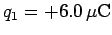is located on the-axis at coordinate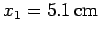. A second particle of charge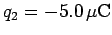is placed on the-axis at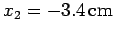. What is the magnitude and direction of the total electrostatic force acting on a third particle of charge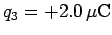placed at the origin (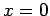)?

Solution: The force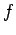acting between charges 1 and 3 is given by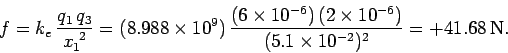Since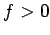, the force is repulsive. This means that the force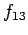exerted by charge 1 on charge 3 is directed along the-axis (i.e., from charge 1 towards charge 3), and is of magnitude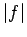. Thus,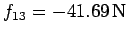. Here, we adopt the convention that forces directed along the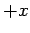-axis are positive, and vice versa. The force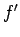acting between charges 2 and 3 is given by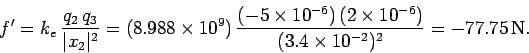Since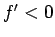, the force is attractive. This means that the force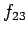exerted by charge 2 on charge 3 is directed along the-axis (i.e., from charge 3 towards charge 2), and is of magnitude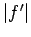. Thus,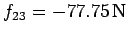.

The resultant force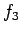acting on charge 3 is the algebraic sum of the forces exerted by charges 1 and 2 separately (the sum is algebraic because all the forces act along the-axis). It follows that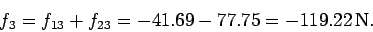Thus, the magnitude of the total force acting on charge 3 is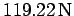, and the force is directed along the-axis (since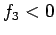).Next: Example 3.2: Electrostatic force between Up: Electricity Previous: Worked Examples
Richard Fitzpatrick 2007-07-14